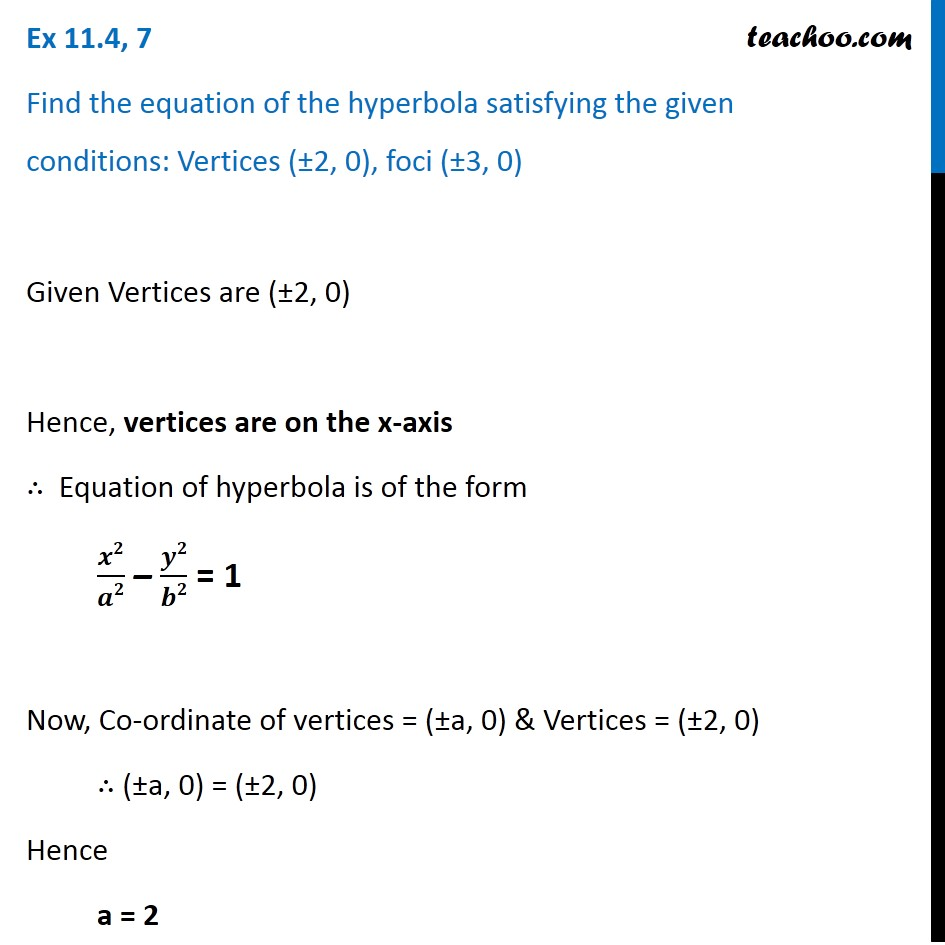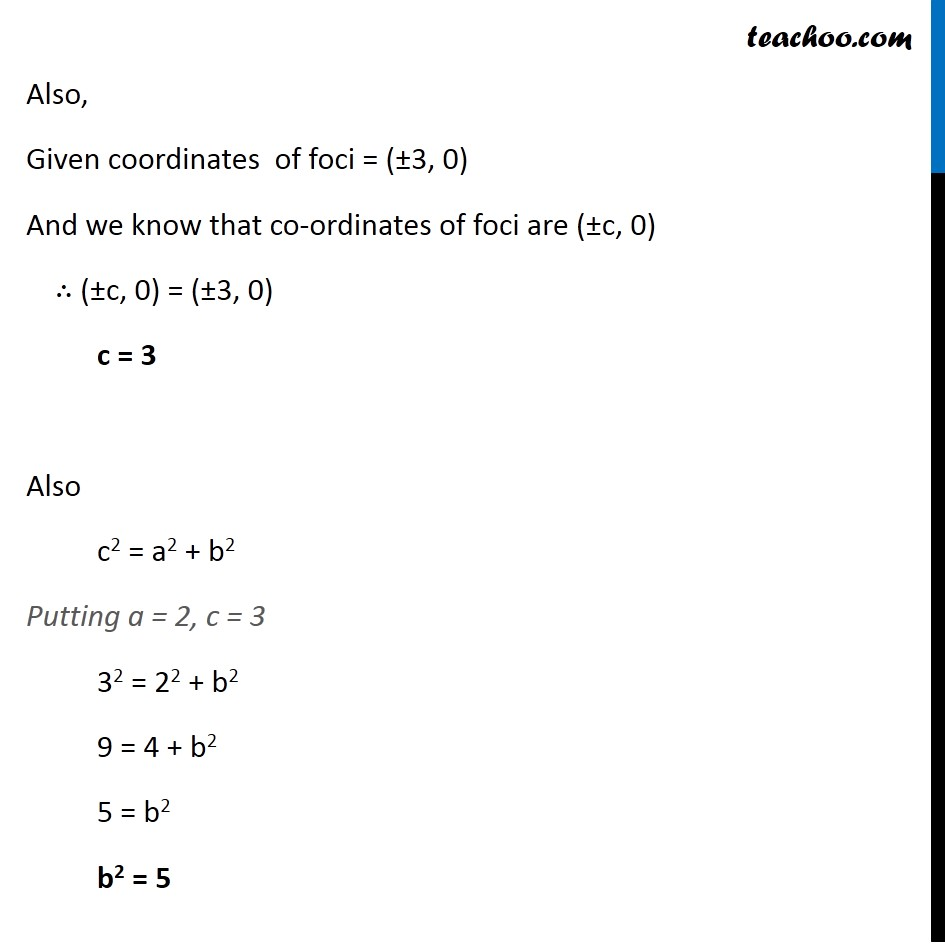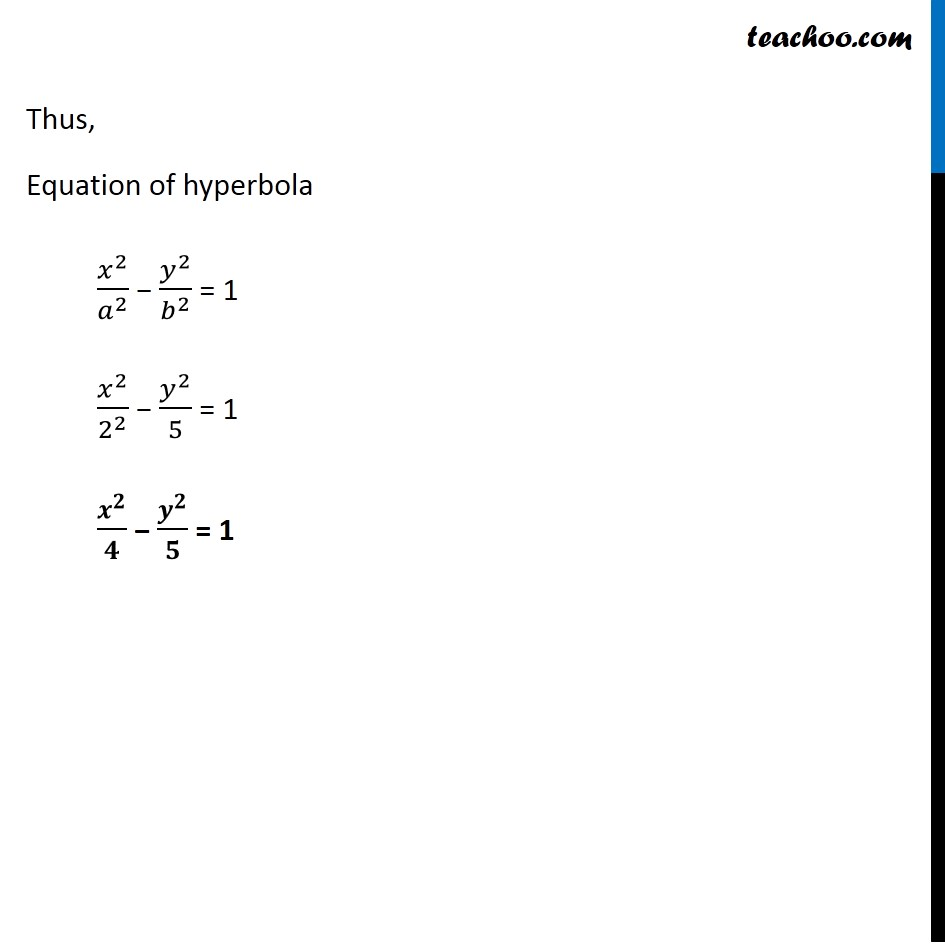Ex 10.4

Chapter 10 Class 11 Conic Sections
Serial order wiseLearn in your speed, with individual attention - Teachoo Maths 1-on-1 Class

### Transcript

Ex 10.4, 7 Find the equation of the hyperbola satisfying the given conditions: Vertices (±2, 0), foci (±3, 0) Given Vertices are (±2, 0) Hence, vertices are on the x-axis ∴ Equation of hyperbola is of the form 𝒙𝟐/𝒂𝟐 – 𝒚𝟐/𝒃𝟐 = 1 Now, Co-ordinate of vertices = (±a, 0) & Vertices = (±2, 0) ∴ (±a, 0) = (±2, 0) Hence a = 2 Also, Given coordinates of foci = (±3, 0) And we know that co-ordinates of foci are (±c, 0) ∴ (±c, 0) = (±3, 0) c = 3 Also c2 = a2 + b2 Putting a = 2, c = 3 32 = 22 + b2 9 = 4 + b2 5 = b2 b2 = 5 Also, Given coordinates of foci = (±3, 0) And we know that co-ordinates of foci are (±c, 0) ∴ (±c, 0) = (±3, 0) c = 3 Also c2 = a2 + b2 Putting a = 2, c = 3 32 = 22 + b2 9 = 4 + b2 5 = b2 b2 = 5 Thus, Equation of hyperbola 𝑥^2/𝑎^2 − 𝑦^2/𝑏^2 = 1 𝑥^2/2^2 − 𝑦^2/5 = 1 𝒙^𝟐/𝟒 − 𝒚^𝟐/𝟓 = 1